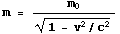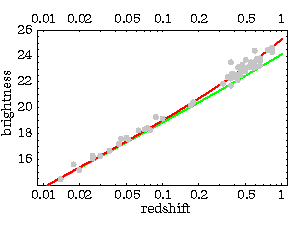# The Standard Hot Big Bang Model of the Universe

## AUTHOR

This document was written in February 2001 by David M. Harrison, Department of Physics, University of Toronto, mailto:harrison@physics.utoronto.ca. This is version 1.3, date (m/d/y) 01/13/02.

## Introduction

This is a brief summary of the Standard Hot Big Bang model of cosmology. It was originally prepared for an upper-year University course in modern Physics with virtually no Mathematics.

You may recall that in 1915 when Einstein was finishing the development of the General Theory of Relativity, the theory's prediction of an expanding universe was in conflict with firm philosophical beliefs of the day. Thus Einstein introduced a fudge factor, the cosmological constant, to force the universe to be static. When in 1929 Hubble showed that the universe really was expanding Einstein immediately dropped the cosmological constant, calling it "the biggest mistake of my life."

Up until the early 1960's, though, a vestige of the idea of a static universe remained in the steady-state model of cosmology. Championed by Sir Fred Hoyle and others, this model posited that although the universe was expanding, matter was being created everywhere in the universe so that the overall density of matter in the universe remains constant. The mechanism for the creation of this matter was never found.

As data began to accumulate on the large scale structure of the universe, the steady-state model began to be in increasing difficulty.

One of the most important of these appeared in 1965 when Penzias and Wilson at AT&T Bell Labs discovered an all pervasive isotropic microwave radiation, corresponding to what would be emitted by a body with a temperature of -270 0C. This radiation is widely interpreted to be a remnant of the big bang, and is usually called the cosmic microwave background radiation.

After Penzias and Wilson's work, the Standard Hot Big Bang model described here "carried the day" and little more was heard from the proponents of the steady-state model.

## The Standard Hot Big Bang Model

There was a big bang some 15 billion years ago, when the size of the universe was zero and the temperature was infinite. The universe then started expanding at near light speed.

The sequence of events in this model is:

Time t = 0 (about 15 billion years ago)
Temperature T = Infinite.
Density = mass per volume = Infinite.
t = 0.01 seconds
T = 100,0.00,000,000 0C.
t = 2 seconds
T = 10,000,000,000 0C.
Density = 100 million kg per cubic meter.
Proton-antiproton and neutron-antineutron pairs begin forming.
t = 3 minutes
T = 1,000,000,000 0C.
Protons and neutrons begin forming hydrogen and helium.
t = 20 minutes
About 25% of the protons and neutrons in the universe are now helium.
t = 10,000 years
T = 10,000 0C.
Density = 0.000,000,000,000,000,01 kg per cubic meter.
Most energy is now mass, not radiation.
Condensation into stars begins. A photograph from the Hubble space telescope of a birthplace of stars appears below.
t = 15 billion years (now)
T = -270 0C. (This temperature is from the Penzias and Wilson experiment described above.)
Density = 10-27 kg per cubic meter.Figure source: http://www.seds.org/hst/M16WF2.html.

In the Standard Hot Big Bang model, each part of the mass-energy of the universe is gravitationally attracted by all the other mass-energy of the universe, so the rate of expansion is expected to be slowing down.

A crucial question was whether this decrease in the rate of expansion is sufficiently great that at some point the expansion would stop and reverse.

If yes, then at some point in the future the size of the universe will again be zero with infinite density and temperature, the Big Crunch. We call such a universe closed. In this case the geometry of the spacetime is similar to the surface of a sphere.

If no, the universe will expand forever. We call such a universe open. In this case the geometry is similar to the surface of a saddle.

The data for the long term fate of the universe is too close to call. Put another way, the geometry of the universe is almost flat.

A variation of Big Bang cosmology is called the Bang-Bang-Bang one. If the universe is closed it will end in a Big Crunch. But the conditions of the Big Crunch are identical to the conditions of the Big Bang. Thus the end of this cycle of the universe is the beginning of the next.

In the early 1960's Huston Smith had an interview with the Dalai Lama. The Dalai Lama asked about the current status of scientific cosmological theories. Smith outlined the Steady State model, the Big Bang model, and the Bang-Bang-Bang one. The Dalai Lama remarked that of the three, the last one was closest to correct. Here is one of my favorite images of a Bang-Bang-Bang cosmology.

"O King of Gods. I have known the dreadful dissolution of the universe. I have seen all perish, again and again, at the end of every cycle. At that terrible time, every single atom dissolves into the primal pure waters of eternity, whence originally all arose. Everything then goes back into the fathomless, wild infinity of the ocean, which is covered with utter darkness and is empty of every sign of animate being. Ah, who will count the universes that have passed away, or the creations that have risen afresh, again and again, from the formless abyss of the vast waters? Who will number the passing ages of the world, as they follow each other endlessly? And who will search through the wide infinities of space to count the universes side by side, each containing its Brahma, its Vishnu, and its Shiva?"

-- Vishnu, in the Brahmavaivarta Purana from H. Zimmer, Myths and Symbols in Indian Art and Civilization, pg. 5.

You should know that the moment of the Big Bang itself presents problems for physicists. The problem is that the language that we use to describe the universe, i.e. mathematics, breaks down when things become zero (the size of the universe) and infinite(the temperature and density). These conditions are called singularities. The mathematics works fine for any time after the Big Bang but not for moment of the bang itself.A slightly mathematical example from the Special Theory of Relativity of a singularity may be helpful. The equation to the right expresses the mass m that a body has when it is moving at a speed v relative to us. The quantity m0 is called the rest mass and is the mass the body has when it is at rest relative to us.

However, if we try to apply this equation to a light particle, a photon, its speed v relative to us is exactly equal to c. Thus, the denominator is the square root of 1 - 1 = 0. In mathematics if we divide any finite quantity by zero the result is infinite. However, for light we say that the rest mass is zero, which is reasonable since we can never have light at rest relative to us.

The mathematicians say that zero divided by zero is undefined, by which they mean you can't do it. Physicists, however, are not bound by the strict rules of mathematics and will happily bend those rules to describe a phenomena. Here we say that the rest mass of zero and the denominator, also zero, work out to be just the finite mass of a photon. Thus we sort of wave our hands and work around this singularity presented by Special Relativity.

I sometimes make an analogy between the way the physicists sometimes bend the rules of mathematics to describe a physical circumstance and the way the James Joyce bent the rules of the English language to describe some inner state.

In a Bang-Bang-Bang cosmology, the singularity of the Big Crunch means that predicting the nature of the next cycle of an expanding universe is impossible. In fact, if one starts going through our physical principles, conservation of energy, three spatial dimensions and one time dimension, etc. etc. it seems that not a single one necessarily will survive into the next cycle.

We shall discover a nearly identical problem with singularities when we discuss Black Holes. There the singularity in its centre similarly wipes all almost all properties of the objects that caused this gravitational collapse.

## Problems

Up until about a decade ago most people accepted the Standard Hot Big Bang model of cosmology. It accounted for a great deal of data regarding the large scale structure of the universe. Some problems were known, and then the list started to grow. These included:

• The flatness problem: Why is the matter density of the universe so close to the unstable critical value between perpetual expansion and recollapse into a Big Crunch?
• The horizon problem: Why does the universe look the same in all directions when it arises out of causally disconnected regions? This problem is most acute for the very smooth cosmic microwave background radiation.
• The dark matter problem: Of what stuff is the Universe predominantly made? Analysis of the gravitational interactions of galaxies shows much more matter than we can see. Nucleosynthesis calculations suggest that this dark matter of the Universe does not consist of ordinary matter - neutrons and protons?Then in 1998 Perlmutter et al. published data that showed that, contrary to expectations, the rate of expansion of the universe is actually increasing. They measured the brightness and redshift of supernovae. The brightness is a direct measure of their distance away from us, and the redshift measures the speed of the supernovae away from us. Thus Perlmutter was taking the same sort of data as Hubble did 70 years before, but this time for supernova, which are much further away from us then the Cepheid variable stars that Hubble used.

The lower line, green for those viewing this in color, corresponds to a closed universe with a rate of expansion decreasing; the upper red line is for a finite universe whose rate of expansion is increasing. The data seem to be more consistent with the upper line.

On should beware, however, that this data was very difficult to collect, and the final numbers are the result of many corrections, calibrations, and calculations. Attempts to replicate these results with greater accuracy and precision are currently under way.

Figure source: http://www.1.slb.com/seed/watch/cosmos/quantum.htm

When this work was published, both Science and the New Scientist magazines called this work the most important of the year.

The Perlmutter data plus other studies have caused some cosmologists to re-introduce a cosmological constant, in this case to cause the rate of expansion to increase.

Finally, up until about 10 years ago I used to state my hope that the question of whether the universe is open or closed would be experimentally answered in the foreseeable future. This hope is now dimmer. Similarly but more optimistically, in about 1988 Stephen Hawking stated the he expected to have a "theory of everything" within 10 years; in 1998 in Toronto he then said that he expected to have a theory of everything within 10 years, but that "the 10 years starts now."